0
178

# Puzzles Questions for IBPS RRB and IBPS PO Prelims

Instructions

Thirteen boxes A, B, C, D, E, F, G, H, I, J, K, L and M are arranged one above the other but necessarily in the same order.
Six boxes are placed between box J and box I. Two boxes are placed between box K and box H. Both boxes B and C are not placed immediately above and below L. Box E is placed above box F but placed below box G. Two boxes are placed between box A and box M. Less than three boxes are placed below box K. One box is placed between box A and box D. Number of boxes placed below box C is equal to the number of boxes placed above box B. One box is placed between box C and box M. Box D is placed below box A. Both boxes C and B are not placed at the bottom of the stack. Number of boxes placed above H is equal to the number of boxes below L. Three boxes are placed between box L and box J.

Question 1: Which of the following statements is true?
i) Number of boxes placed above box J is equal to the number of boxes placed below box M
ii) Three boxes are placed between box A and box I
iii) Number of boxes placed between box C and box E is equal to the number of boxes placed between box J and box A

a) Only i

b) Only iii

c) Only i and ii

d) Only i and iii

e) All i, ii and iii

Solution:

Less than three boxes are placed below box K. Two boxes are placed between box K and box H. Number of boxes placed above H is equal to the number of boxes below L. The three possibilities are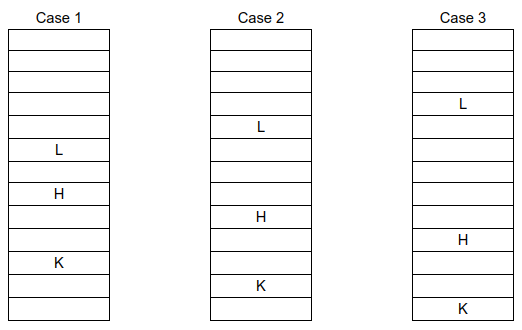Three boxes are placed between box L and box J. Six boxes are placed between box J and box I. J is possible in two places in Case 1 which are represented as Case 1a and Case 1b.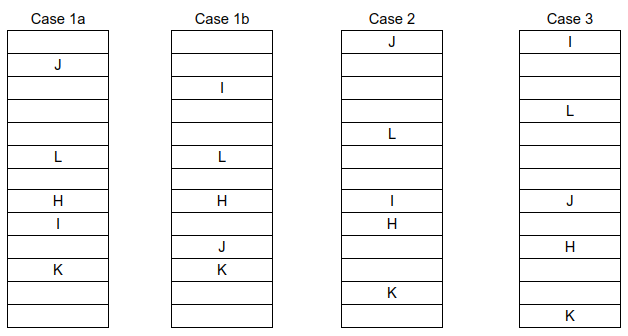Number of boxes placed below box C is equal to the number of boxes placed above box B. Both boxes B and C are not placed immediately above and below L. Both boxes C and B are not placed at the bottom of the stack.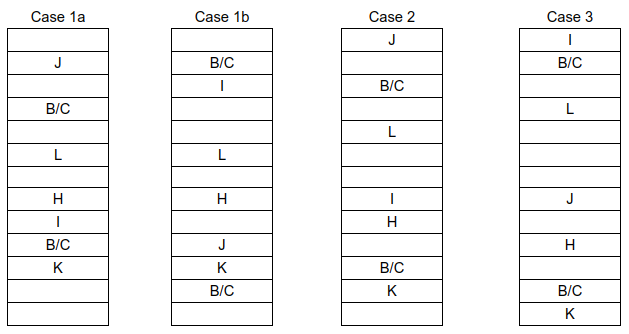One box is placed between box C and box M. Two boxes are placed between box A and box M. One box is placed between box A and box D. Box D is placed below box A. M is not possible in Case 3. Hence Case 3 is eliminated. A and D are not possible in Case 1a and Case 2. Hence Case 1a and Case 2 are eliminated.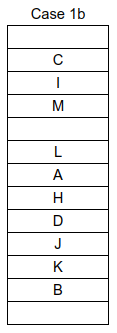Box E is placed above box F but placed below box G.
The final arrangement is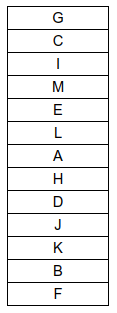Hence, the correct answer is Option E

Question 2: If all the boxes are arranged in alphabetical order from top to bottom then the position of how many boxes remain unchanged?

a) Three

b) Five

c) Six

d) Four

e) Two

Solution:

Less than three boxes are placed below box K. Two boxes are placed between box K and box H. Number of boxes placed above H is equal to the number of boxes below L. The three possibilities areThree boxes are placed between box L and box J. Six boxes are placed between box J and box I. J is possible in two places in Case 1 which are represented as Case 1a and Case 1b.Number of boxes placed below box C is equal to the number of boxes placed above box B. Both boxes B and C are not placed immediately above and below L. Both boxes C and B are not placed at the bottom of the stack.One box is placed between box C and box M. Two boxes are placed between box A and box M. One box is placed between box A and box D. Box D is placed below box A. M is not possible in Case 3. Hence Case 3 is eliminated. A and D are not possible in Case 1a and Case 2. Hence Case 1a and Case 2 are eliminated.Box E is placed above box F but placed below box G.
The final arrangement is$\therefore$ If all the boxes are arranged in alphabetical order from top to bottom then the position of E, H, J and K boxes remain unchanged.
Hence, the correct answer is Option D

Question 3: How many boxes are placed between box G and box D?

a) Five

b) Nine

c) Six

d) Seven

e) Eight

Solution:

Less than three boxes are placed below box K. Two boxes are placed between box K and box H. Number of boxes placed above H is equal to the number of boxes below L. The three possibilities areThree boxes are placed between box L and box J. Six boxes are placed between box J and box I. J is possible in two places in Case 1 which are represented as Case 1a and Case 1b.Number of boxes placed below box C is equal to the number of boxes placed above box B. Both boxes B and C are not placed immediately above and below L. Both boxes C and B are not placed at the bottom of the stack.One box is placed between box C and box M. Two boxes are placed between box A and box M. One box is placed between box A and box D. Box D is placed below box A. M is not possible in Case 3. Hence Case 3 is eliminated. A and D are not possible in Case 1a and Case 2. Hence Case 1a and Case 2 are eliminated.Box E is placed above box F but placed below box G.
The final arrangement is$\therefore$ Seven boxes are placed between box G and box D.
Hence, the correct answer is Option D

Instructions

Study the information given below and answer the given questions.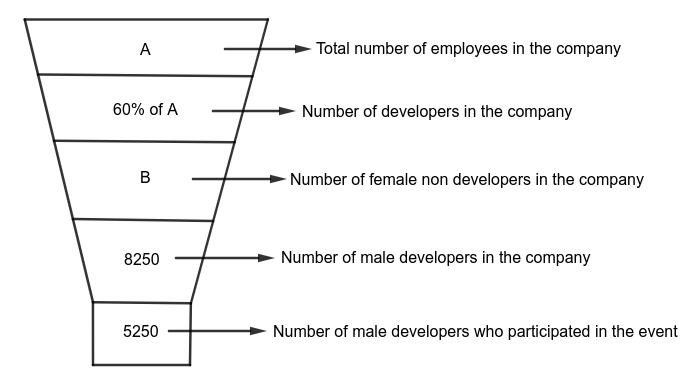Note:
i) Total number of employees in the company = Number of developers in the company + Number of non developers in the company.
ii) Number of developers in the company = Number of male developers in the company + Number of female developers in the company.
iii) Number of non developers in the company = Number of male non developers in the company + Number of female non developers in the company.
iv) Number of male developers in the company = Number of male developers who participated in the event + Number of male developers who didn’t participate in the event.
v) Number of female non developers in the company = Number of female non developers who participated in the event + Number of female non developers who didn’t participate in the event.
vi) Number of female non developers who didn’t participate in the event is 28.57% less than the number of female non developers who participated in the event.
vii) Number of female developers in the company is 1950 more than the number of female non developers in the company.
viii) The ratio of the number of male developers who didn’t participate in the event and the number of female non developers who participated in the event is 15:14 respectively.

Question 4: Out of the female non developers who participated in the event $\frac{2}{7}$th participated in singing, $\frac{1}{7}$th participated in dancing and the remaining participated in games then what is the number of female non developers who participated in games?

a) 400

b) 1600

c) 800

d) 1200

e) None of the above

Solution:

Number of male developers in the company = 8250
Number of male developers who participated in the event = 5250
Number of male developers who didn’t participate in the event = 8250 – 5250
= 3000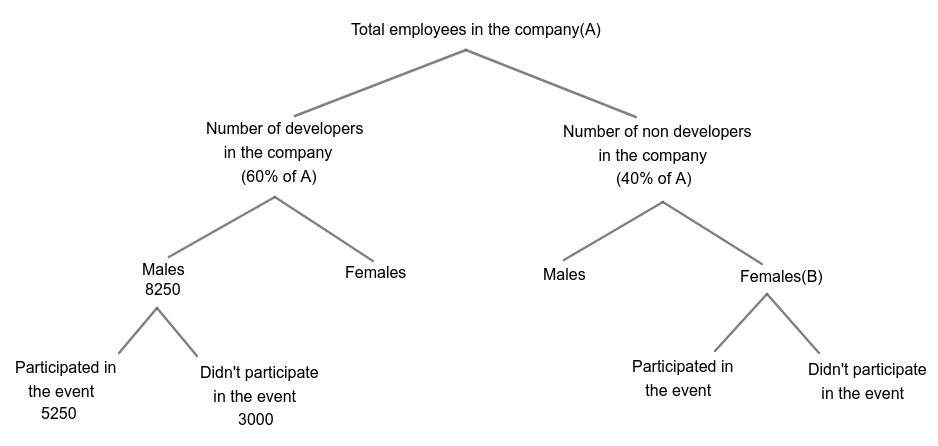The ratio of the number of male developers who didn’t participate in the event and the number of female non developers who participated in the event is 15:14 respectively.
Number of female non developers who participated in the event = $\frac{14}{15}\times$3000
= 2800

Number of female non developers who didn’t participate in the event is 28.57% less than the number of female non developers who participated in the event.
Number of female non developers who didn’t participate in the event = 2800 – $\frac{2}{7}\times$2800
= 2800 – 800
= 2000

Number of female non developers in the company(B) = 2800 + 2000 = 4800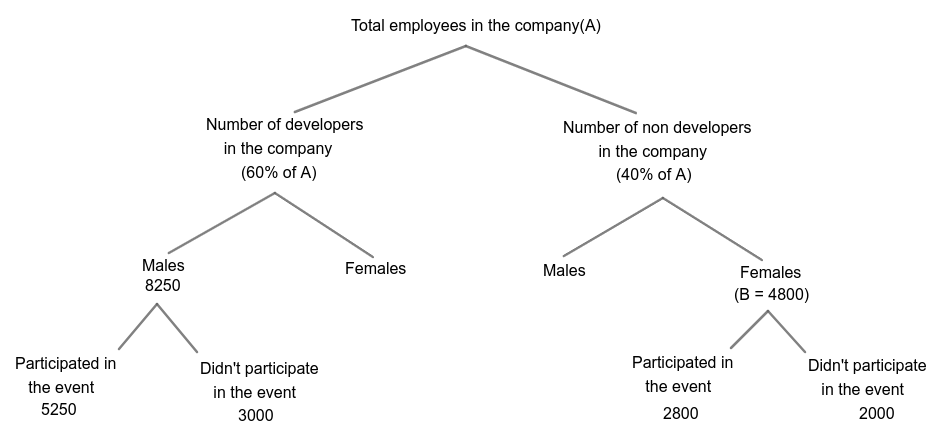Number of female developers in the company is 1950 more than the number of female non developers in the company.
Number of female developers in the company = 4800 + 1950
= 6750

Number of developers in the company = Number of male developers + Number of female developers
= 8250 + 6750
= 15000
60% of A = 15000
A = 25000

Total number of employees in the company(A) = 25000
Number of non developers in the company = 40% of A
= 40% of 25000
= 10000

Number of male non developers in the company = Number of non developers in the company – Number of female non developers in the company
= 10000 – 4800
= 5200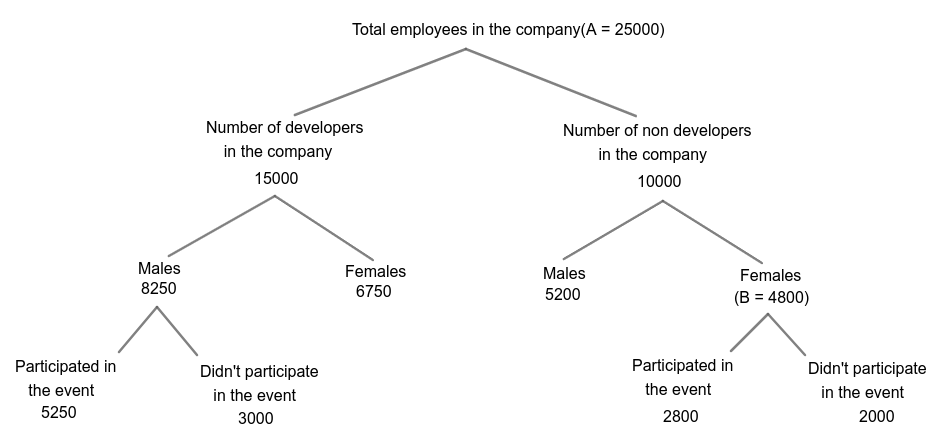Out of the female non developers who participated in the event $\frac{2}{7}$th participated in singing, $\frac{1}{7}$th participated in dancing and the remaining participated in games.
Number of female non developers who participated in games = (1-$\frac{2}{7}$-$\frac{1}{7}$) x 2800
= $\frac{4}{7}$ x 2800
= 1600
Hence, the correct answer is Option B

Question 5: If the number of female developers who participated in the event is 1350 more than the number of female developers who didn’t participate in the event then what is the ratio of the number of male developers who didn’t participate in the event and the number of female developers who didn’t participate in the event respectively?

a) 10 : 9

b) 9 : 8

c) 8 : 9

d) 9 : 10

e) None of the above

Solution:

Number of male developers in the company = 8250
Number of male developers who participated in the event = 5250
Number of male developers who didn’t participate in the event = 8250 – 5250
= 3000The ratio of the number of male developers who didn’t participate in the event and the number of female non developers who participated in the event is 15:14 respectively.
Number of female non developers who participated in the event = $\frac{14}{15}\times$3000
= 2800

Number of female non developers who didn’t participate in the event is 28.57% less than the number of female non developers who participated in the event.
Number of female non developers who didn’t participate in the event = 2800 – $\frac{2}{7}\times$2800
= 2800 – 800
= 2000

Number of female non developers in the company(B) = 2800 + 2000 = 4800Number of female developers in the company is 1950 more than the number of female non developers in the company.
Number of female developers in the company = 4800 + 1950
= 6750

Number of developers in the company = Number of male developers + Number of female developers
= 8250 + 6750
= 15000
60% of A = 15000
A = 25000

Total number of employees in the company(A) = 25000
Number of non developers in the company = 40% of A
= 40% of 25000
= 10000

Number of male non developers in the company = Number of non developers in the company – Number of female non developers in the company
= 10000 – 4800
= 5200The number of female developers who participated in the event is 1350 more than the number of female developers who didn’t participate in the event.
Let the number of female developers who didn’t participate in the event = p
Number of female developers who participated in the event = p + 1350

Number of female developers = 6750
p + 1350 + p = 6750
2p = 5400
p = 2700
Number of female developers who didn’t participate in the event = p = 2700

Required ratio = 3000 : 2700
= 10 : 9
Hence, the correct answer is Option A

Question 6: If 54.54% of the number of male developers are graduates then what is the number of male developers who are not graduates?

a) 4500

b) 3950

c) 3600

d) 3750

e) None of the above

Solution:

Number of male developers in the company = 8250
Number of male developers who participated in the event = 5250
Number of male developers who didn’t participate in the event = 8250 – 5250
= 3000The ratio of the number of male developers who didn’t participate in the event and the number of female non developers who participated in the event is 15:14 respectively.
Number of female non developers who participated in the event = $\frac{14}{15}\times$3000
= 2800

Number of female non developers who didn’t participate in the event is 28.57% less than the number of female non developers who participated in the event.
Number of female non developers who didn’t participate in the event = 2800 – $\frac{2}{7}\times$2800
= 2800 – 800
= 2000

Number of female non developers in the company(B) = 2800 + 2000 = 4800Number of female developers in the company is 1950 more than the number of female non developers in the company.
Number of female developers in the company = 4800 + 1950
= 6750

Number of developers in the company = Number of male developers + Number of female developers
= 8250 + 6750
= 15000
60% of A = 15000
A = 25000

Total number of employees in the company(A) = 25000
Number of non developers in the company = 40% of A
= 40% of 25000
= 10000

Number of male non developers in the company = Number of non developers in the company – Number of female non developers in the company
= 10000 – 4800
= 520054.54% of the number of male developers are graduates.
Number of male developers who are graduates = $\frac{6}{11}\times$8250
= 4500

Number of male developers who are not graduates = 8250 – 4500
= 3750
Hence, the correct answer is Option D

Question 7: The number of female non developers who didn’t participate in the event is what percent of the number of non developers in the company?

a) 22%

b) 12%

c) 16%

d) 24%

e) None of the above

Solution:

Number of male developers in the company = 8250
Number of male developers who participated in the event = 5250
Number of male developers who didn’t participate in the event = 8250 – 5250
= 3000The ratio of the number of male developers who didn’t participate in the event and the number of female non developers who participated in the event is 15:14 respectively.
Number of female non developers who participated in the event = $\frac{14}{15}\times$3000
= 2800

Number of female non developers who didn’t participate in the event is 28.57% less than the number of female non developers who participated in the event.
Number of female non developers who didn’t participate in the event = 2800 – $\frac{2}{7}\times$2800
= 2800 – 800
= 2000

Number of female non developers in the company(B) = 2800 + 2000 = 4800Number of female developers in the company is 1950 more than the number of female non developers in the company.
Number of female developers in the company = 4800 + 1950
= 6750

Number of developers in the company = Number of male developers + Number of female developers
= 8250 + 6750
= 15000
60% of A = 15000
A = 25000

Total number of employees in the company(A) = 25000
Number of non developers in the company = 40% of A
= 40% of 25000
= 10000

Number of male non developers in the company = Number of non developers in the company – Number of female non developers in the company
= 10000 – 4800
= 5200Required percentage = $\frac{2000}{10000}\times$100
= 20%
Hence, the correct answer is Option E

Question 8: What is the value of ‘A’?

a) 32000

b) 28000

c) 30000

d) 25000

e) None of the above

Solution:

Number of male developers in the company = 8250
Number of male developers who participated in the event = 5250
Number of male developers who didn’t participate in the event = 8250 – 5250
= 3000The ratio of the number of male developers who didn’t participate in the event and the number of female non developers who participated in the event is 15:14 respectively.
Number of female non developers who participated in the event = $\frac{14}{15}\times$3000
= 2800

Number of female non developers who didn’t participate in the event is 28.57% less than the number of female non developers who participated in the event.
Number of female non developers who didn’t participate in the event = 2800 – $\frac{2}{7}\times$2800
= 2800 – 800
= 2000

Number of female non developers in the company(B) = 2800 + 2000 = 4800Number of female developers in the company is 1950 more than the number of female non developers in the company.
Number of female developers in the company = 4800 + 1950
= 6750

Number of developers in the company = Number of male developers + Number of female developers
= 8250 + 6750
= 15000
60% of A = 15000
A = 25000

Total number of employees in the company(A) = 25000
Number of non developers in the company = 40% of A
= 40% of 25000
= 10000

Number of male non developers in the company = Number of non developers in the company – Number of female non developers in the company
= 10000 – 4800
= 5200Hence, the correct answer is Option D

Question 9: What is the difference between the number of female developers and the number of male non developers?

a) 1650

b) 1550

c) 1750

d) 1950

e) None of the above

Solution:

Number of male developers in the company = 8250
Number of male developers who participated in the event = 5250
Number of male developers who didn’t participate in the event = 8250 – 5250
= 3000The ratio of the number of male developers who didn’t participate in the event and the number of female non developers who participated in the event is 15:14 respectively.
Number of female non developers who participated in the event = $\frac{14}{15}\times$3000
= 2800

Number of female non developers who didn’t participate in the event is 28.57% less than the number of female non developers who participated in the event.
Number of female non developers who didn’t participate in the event = 2800 – $\frac{2}{7}\times$2800
= 2800 – 800
= 2000

Number of female non developers in the company(B) = 2800 + 2000 = 4800Number of female developers in the company is 1950 more than the number of female non developers in the company.
Number of female developers in the company = 4800 + 1950
= 6750

Number of developers in the company = Number of male developers + Number of female developers
= 8250 + 6750
= 15000
60% of A = 15000
A = 25000

Total number of employees in the company(A) = 25000
Number of non developers in the company = 40% of A
= 40% of 25000
= 10000

Number of male non developers in the company = Number of non developers in the company – Number of female non developers in the company
= 10000 – 4800
= 5200The difference between the number of female developers and the number of male non developers = 6750 – 5200
= 1550
Hence, the correct answer is Option B

Instructions

Six people A, B, C, D, E and F are working on six different posts namely CEO (Chief Executive Officer), GM (General Manager), DGM (Deputy General Manager), AGM (Assistant General Manager), CM (Chief Manager) and SM (Senior Manager) of an organization. Each of them likes different sweets namely Jalebi, Laddu, Ghewar, Peda, Barfi and Kheer. Each of them has a different lucky number from 1 to 9. CEO is the senior most position and SM is the junior most position in the organization. Lucky number of the one who likes Ghewar is one more than the lucky number of the one who likes Barfi. The one who likes Jalebi is senior to B. The difference between the lucky number of the one who likes Peda and the one who likes Kheer is three. The sum of the lucky numbers of C and D is the multiple of seven. The one who is on the post of CM does not like Laddu. More than two people are senior to E who does not like Kheer. D is not in the post of CEO. The lucky number of A is not three. The number of people junior to the one who likes Ghewar is the same as the number of people senior to the one who likes Jalebi. Neither C nor B like Barfi. Lucky number of the one who is on the post of CEO is a square number. D is not junior to A. The number of people senior to E is one more than the number of people junior to the one who likes Ghewar. The one who likes Laddu is junior to the one who likes Peda but senior to C who is not on the post of DGM. The sum of the lucky numbers of D and B is the multiple of five. Lucky number of the one who likes Jalebi is not an odd number.

Question 10: Which of the following statements are not true?
(i) The sum of the lucky numbers of all the six people is the multiples of 6.
(ii) If all the people are arranged as per the alphabetical order from senior to junior posts respectively, then the post of only one person remains unchanged.
(iii) C likes Barfi.

a) Only (i) and (ii)

b) Only (i) and (iii)

c) Only (ii) and (iii)

d) Only (iii)

e) Only (ii)

Solution:

More than two people are senior to E who does not like Kheer. The number of people senior to E is one more than the number of people junior to the one who likes Ghewar. The number of people junior to the one who likes Ghewar is the same as the number of people senior to the one who likes Jalebi.
As per the above given conditions, there are three possible cases which are given below in Case(i), Case(ii) and Case(iii).The one who likes Jalebi is senior to B.
In Case(iii), B is not possible as per the above given condition. So this case is eliminated.
In Case(i), B is possible in two places which are given below in Case(i)(a) and Case(i)(b).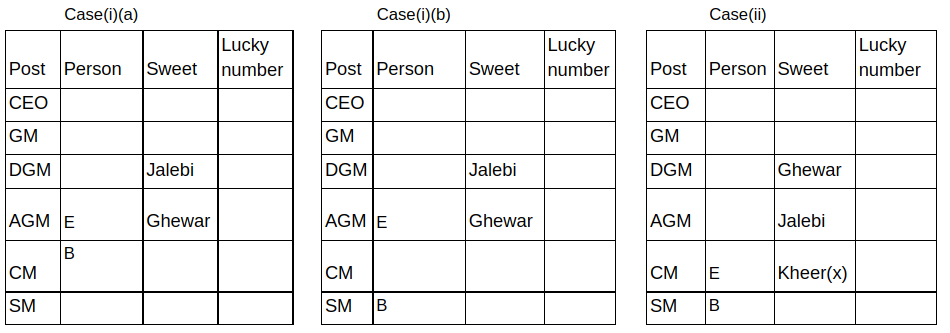The one who likes Laddu is junior to the one who likes Peda but senior to C who is not on the post of DGM. The one who is on the post of CM does not like Laddu.
In Case(i)(b), C is not possible as per the above given condition. So this case is eliminated.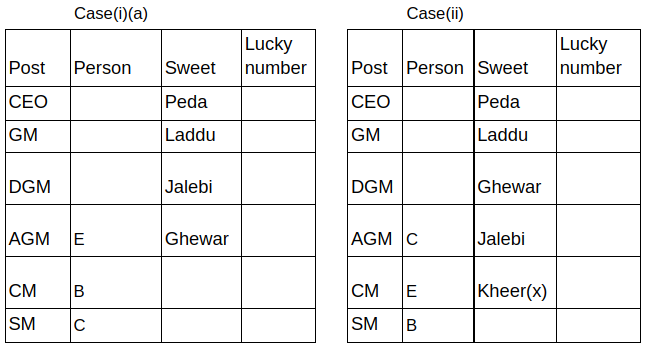Neither C nor B like Barfi. D is not in the post of CEO. D is not junior to A.
In Case(i)(a), Barfi is not possible as per the above given condition. So this case is eliminated.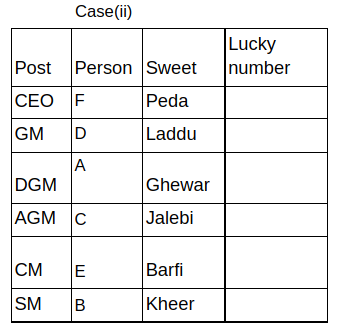Lucky number of the one who is on the post of CEO is a square number. The difference between the lucky number of the one who likes Peda and the one who likes Kheer is three.
So the lucky number of F can be 1, 4 and 9 which are given below in Case(ii)(a), Case(ii)(b) and Case(ii)(c).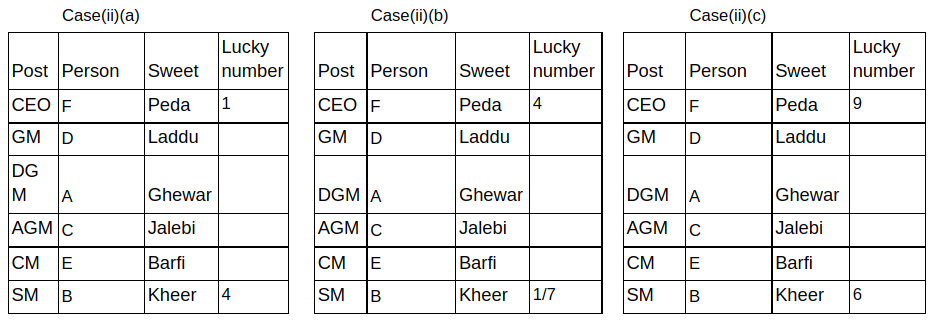The sum of the lucky numbers of D and B is the multiple of five.
In Case(ii)(a), B = 4, then D = 6.
In Case(ii)(b), when B = 1, then D = 9 and when B = 7, then D = 8.
In Case(ii)(c), B = 6, then D = 4.
In each case other values are not possible as per the given condition.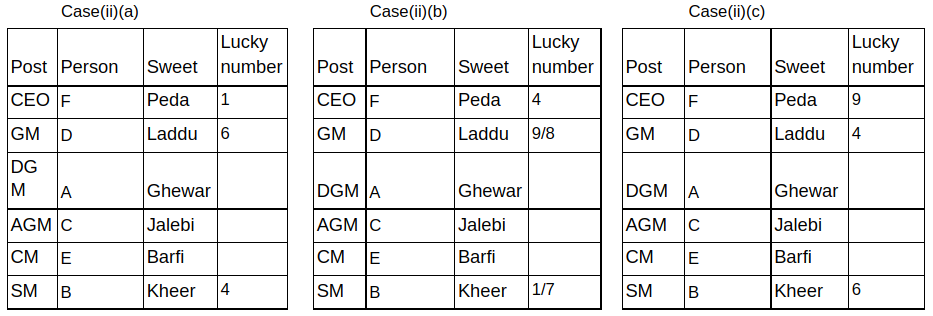The sum of the lucky numbers of C and D is the multiple of seven.
In Case(ii)(a), D = 6, then C = 8.
In Case(ii)(b), when D = 9, then C = 5 and when D = 8, then C = 6.
In Case(ii)(c), D = 4, then C = 3.
In each case other values are not possible as per the given condition.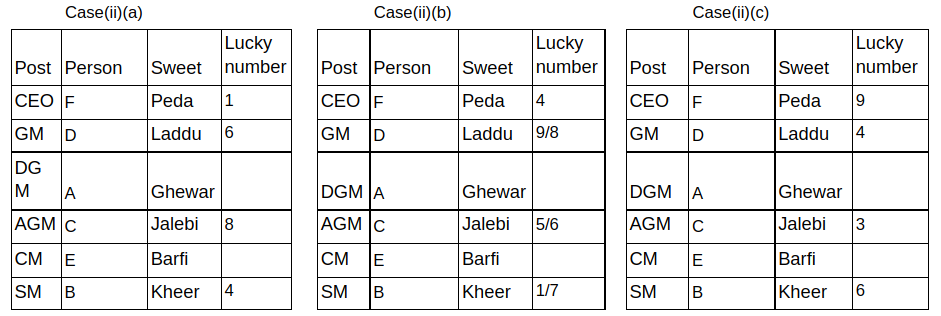Lucky number of the one who likes Jalebi is not an odd number.
In Case(ii)(c), the above given condition is not possible. So this case is eliminated.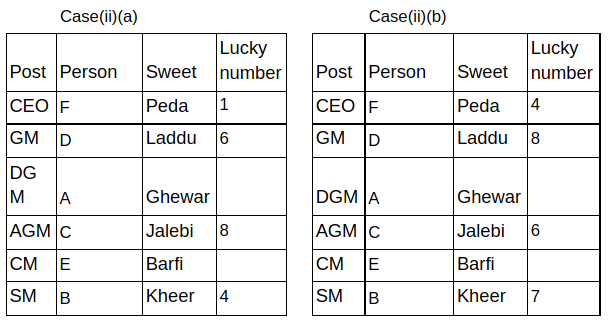The lucky number of A is not three. Lucky number of the one who likes Ghewar is one more than the lucky number of the one who likes Barfi.
In Case(ii)(a), the above given condition is not possible. So this case is eliminated.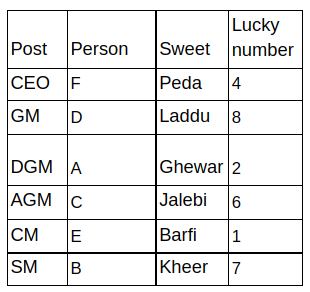Therefore, we got the final arrangement which is given above.
Hence, option b is the correct answer.

Question 11: The number of people junior to the one who likes Kheer is _____ less than the number of people senior to the one whose lucky number is ___.

a) one, 6

b) one, 8

c) two, 1

d) two, 4

e) None of the above

Solution:

More than two people are senior to E who does not like Kheer. The number of people senior to E is one more than the number of people junior to the one who likes Ghewar. The number of people junior to the one who likes Ghewar is the same as the number of people senior to the one who likes Jalebi.
As per the above given conditions, there are three possible cases which are given below in Case(i), Case(ii) and Case(iii).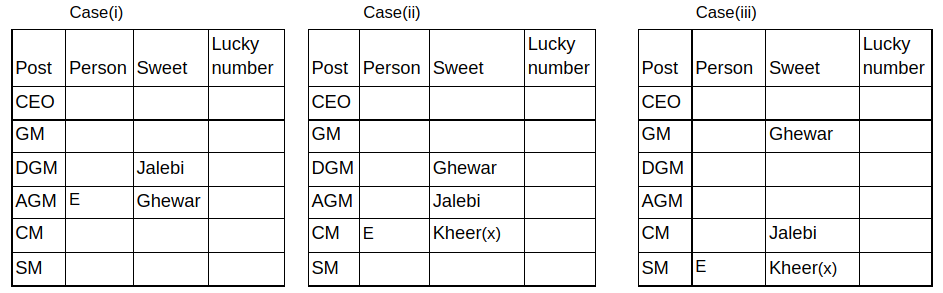The one who likes Jalebi is senior to B.
In Case(iii), B is not possible as per the above given condition. So this case is eliminated.
In Case(i), B is possible in two places which are given below in Case(i)(a) and Case(i)(b).The one who likes Laddu is junior to the one who likes Peda but senior to C who is not on the post of DGM. The one who is on the post of CM does not like Laddu.
In Case(i)(b), C is not possible as per the above given condition. So this case is eliminated.Neither C nor B like Barfi. D is not in the post of CEO. D is not junior to A.
In Case(i)(a), Barfi is not possible as per the above given condition. So this case is eliminated.Lucky number of the one who is on the post of CEO is a square number. The difference between the lucky number of the one who likes Peda and the one who likes Kheer is three.
So the lucky number of F can be 1, 4 and 9 which are given below in Case(ii)(a), Case(ii)(b) and Case(ii)(c).The sum of the lucky numbers of D and B is the multiple of five.
In Case(ii)(a), B = 4, then D = 6.
In Case(ii)(b), when B = 1, then D = 9 and when B = 7, then D = 8.
In Case(ii)(c), B = 6, then D = 4.
In each case other values are not possible as per the given condition.The sum of the lucky numbers of C and D is the multiple of seven.
In Case(ii)(a), D = 6, then C = 8.
In Case(ii)(b), when D = 9, then C = 5 and when D = 8, then C = 6.
In Case(ii)(c), D = 4, then C = 3.
In each case other values are not possible as per the given condition.Lucky number of the one who likes Jalebi is not an odd number.
In Case(ii)(c), the above given condition is not possible. So this case is eliminated.The lucky number of A is not three. Lucky number of the one who likes Ghewar is one more than the lucky number of the one who likes Barfi.
In Case(ii)(a), the above given condition is not possible. So this case is eliminated.Therefore, we got the final arrangement which is given above.

According to the above arrangement, the number of people junior to the one who likes Kheer is one less than the number of people senior to the one whose lucky number is 8.
Hence, option b is the correct answer.

Question 12: The one whose lucky number is 3 is on which of the following posts?

a) GM

b) CM

c) SM

d) AGM

e) None

Solution:

More than two people are senior to E who does not like Kheer. The number of people senior to E is one more than the number of people junior to the one who likes Ghewar. The number of people junior to the one who likes Ghewar is the same as the number of people senior to the one who likes Jalebi.
As per the above given conditions, there are three possible cases which are given below in Case(i), Case(ii) and Case(iii).The one who likes Jalebi is senior to B.
In Case(iii), B is not possible as per the above given condition. So this case is eliminated.
In Case(i), B is possible in two places which are given below in Case(i)(a) and Case(i)(b).The one who likes Laddu is junior to the one who likes Peda but senior to C who is not on the post of DGM. The one who is on the post of CM does not like Laddu.
In Case(i)(b), C is not possible as per the above given condition. So this case is eliminated.Neither C nor B like Barfi. D is not in the post of CEO. D is not junior to A.
In Case(i)(a), Barfi is not possible as per the above given condition. So this case is eliminated.Lucky number of the one who is on the post of CEO is a square number. The difference between the lucky number of the one who likes Peda and the one who likes Kheer is three.
So the lucky number of F can be 1, 4 and 9 which are given below in Case(ii)(a), Case(ii)(b) and Case(ii)(c).The sum of the lucky numbers of D and B is the multiple of five.
In Case(ii)(a), B = 4, then D = 6.
In Case(ii)(b), when B = 1, then D = 9 and when B = 7, then D = 8.
In Case(ii)(c), B = 6, then D = 4.
In each case other values are not possible as per the given condition.The sum of the lucky numbers of C and D is the multiple of seven.
In Case(ii)(a), D = 6, then C = 8.
In Case(ii)(b), when D = 9, then C = 5 and when D = 8, then C = 6.
In Case(ii)(c), D = 4, then C = 3.
In each case other values are not possible as per the given condition.Lucky number of the one who likes Jalebi is not an odd number.
In Case(ii)(c), the above given condition is not possible. So this case is eliminated.The lucky number of A is not three. Lucky number of the one who likes Ghewar is one more than the lucky number of the one who likes Barfi.
In Case(ii)(a), the above given condition is not possible. So this case is eliminated.Therefore, we got the final arrangement which is given above.

According to the above arrangement, none of the person’s lucky number is 3.
Hence, option e is the correct answer.

Question 13: How many people’s lucky number is a prime number?

a) None

b) One

c) Two

d) Three

e) Four

Solution:

More than two people are senior to E who does not like Kheer. The number of people senior to E is one more than the number of people junior to the one who likes Ghewar. The number of people junior to the one who likes Ghewar is the same as the number of people senior to the one who likes Jalebi.
As per the above given conditions, there are three possible cases which are given below in Case(i), Case(ii) and Case(iii).The one who likes Jalebi is senior to B.
In Case(iii), B is not possible as per the above given condition. So this case is eliminated.
In Case(i), B is possible in two places which are given below in Case(i)(a) and Case(i)(b).The one who likes Laddu is junior to the one who likes Peda but senior to C who is not on the post of DGM. The one who is on the post of CM does not like Laddu.
In Case(i)(b), C is not possible as per the above given condition. So this case is eliminated.Neither C nor B like Barfi. D is not in the post of CEO. D is not junior to A.
In Case(i)(a), Barfi is not possible as per the above given condition. So this case is eliminated.Lucky number of the one who is on the post of CEO is a square number. The difference between the lucky number of the one who likes Peda and the one who likes Kheer is three.
So the lucky number of F can be 1, 4 and 9 which are given below in Case(ii)(a), Case(ii)(b) and Case(ii)(c).The sum of the lucky numbers of D and B is the multiple of five.
In Case(ii)(a), B = 4, then D = 6.
In Case(ii)(b), when B = 1, then D = 9 and when B = 7, then D = 8.
In Case(ii)(c), B = 6, then D = 4.
In each case other values are not possible as per the given condition.The sum of the lucky numbers of C and D is the multiple of seven.
In Case(ii)(a), D = 6, then C = 8.
In Case(ii)(b), when D = 9, then C = 5 and when D = 8, then C = 6.
In Case(ii)(c), D = 4, then C = 3.
In each case other values are not possible as per the given condition.Lucky number of the one who likes Jalebi is not an odd number.
In Case(ii)(c), the above given condition is not possible. So this case is eliminated.The lucky number of A is not three. Lucky number of the one who likes Ghewar is one more than the lucky number of the one who likes Barfi.
In Case(ii)(a), the above given condition is not possible. So this case is eliminated.Therefore, we got the final arrangement which is given above.

According to the above arrangement, two people’s lucky number is a prime number.
Hence, option c is the correct answer.

Instructions

Eight cars A, B, C, D, E, F, G and H parked in a line facing North direction but not necessarily in the respective order. The distance between two adjacent cars are successive multiples of seven(i.e, if the distance between 1st and 2nd car is 7 m then the distance between 2nd and 3rd car is 14 m and the distance between 3rd and 4th car is 21 m so on).
Distance between car F and car A is 42 m. Four cars are parked between car B and car D. Distance between car G and car B is less than 63 m. Car F is placed immediately to the right of A. Two cars are parked between car A and car G. Distance between car B and car G is not less than 28 m. Distance between no adjacent cars is zero. Car C is not adjacent to Car E. Distance between car D and car E is 49 m. Distance between car C and car E is more than 63 m. Car E starts moving 25 m towards South then takes a left turn and moves 42 m to reach point Y. Car B starts moving 40 m towards North and takes a right turn and moves 56 m to reach point Z.

Question 14: What is the direction of car H with respect to the final position of car E?

a) North East

b) South West

c) West

d) North West

e) South East

Solution:

Car F is placed immediately to the right of A. Distance between car F and car A is 42 m. Two cars are placed between car A and car G. The two possibilities are
Case 1: G (21) _ (28) _ (35) A (42) F
Case 2: A (42) F (49) _ (56) G

Distance between car G and car B is less than 63 m. Distance between car B and car G is not less than 28 m. Distance between no adjacent cars is zero.
Case 1: G (21) _ (28) B (35) A (42) F
Case 2: A (42) F (49) B (56) G

Four cars are parked between car B and car D. D is possible in two places in Case 2 which are represented as Case 2a and Case 2b.
Case 1a: G (21) _ (28) B (35) A (42) F (49) _ (56) _ (63) D
Case 2a: D (21) _ (28) _ (35) A (42) F (49) B (56) G
Case 2b: A (42) F (49) B (56) G (63) _ (70) _ (77) _ (84) D

Distance between car D and car E is 49 m. E is not possible in Case 1a and Case 2b. Hence Case 1a and Case 2b are eliminated.
Case 2a: D (21) _ (28) E (35) A (42) F (49) B (56) G

Car C is not adjacent to Car E. Distance between car C and car E is more than 63 m.
The final arrangement is
D (21) H (28) E (35) A (42) F (49) B (56) G (63) C

Car E starts moving 25 m towards South then takes a left turn and moves 42 m. Car B starts moving 40 m towards North and takes a right turn and moves 56 m.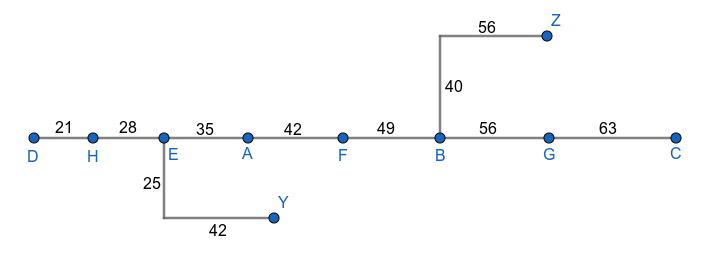$\therefore$ Car H is North West of the final position of car E.
Hence, the correct answer is Option D

Question 15: What is the shortest distance between the initial and final position of car B?

a) 8$\sqrt{74}$

b) 4$\sqrt{74}$

c) 8$\sqrt{37}$

d) 2$\sqrt{37}$

e) 4$\sqrt{37}$

Solution:

Car F is placed immediately to the right of A. Distance between car F and car A is 42 m. Two cars are placed between car A and car G. The two possibilities are
Case 1: G (21) _ (28) _ (35) A (42) F
Case 2: A (42) F (49) _ (56) G

Distance between car G and car B is less than 63 m. Distance between car B and car G is not less than 28 m. Distance between no adjacent cars is zero.
Case 1: G (21) _ (28) B (35) A (42) F
Case 2: A (42) F (49) B (56) G

Four cars are parked between car B and car D. D is possible in two places in Case 2 which are represented as Case 2a and Case 2b.
Case 1a: G (21) _ (28) B (35) A (42) F (49) _ (56) _ (63) D
Case 2a: D (21) _ (28) _ (35) A (42) F (49) B (56) G
Case 2b: A (42) F (49) B (56) G (63) _ (70) _ (77) _ (84) D

Distance between car D and car E is 49 m. E is not possible in Case 1a and Case 2b. Hence Case 1a and Case 2b are eliminated.
Case 2a: D (21) _ (28) E (35) A (42) F (49) B (56) G

Car C is not adjacent to Car E. Distance between car C and car E is more than 63 m.
The final arrangement is
D (21) H (28) E (35) A (42) F (49) B (56) G (63) C

Car E starts moving 25 m towards South then takes a left turn and moves 42 m. Car B starts moving 40 m towards North and takes a right turn and moves 56 m.Shortest distance between initial and final position of car B = $\sqrt{40^2+56^2}$
= $\sqrt{1600+3136}$
= $\sqrt{4736}$
= 8$\sqrt{74}$
Hence, the correct answer is Option A

Question 16: What is the position of car A with respect to car G?

a) 168 m to the left

b) 147 m to the right

c) 168 m to the right

d) 126 m to the right

e) 147 m to the left

Solution:

Car F is placed immediately to the right of A. Distance between car F and car A is 42 m. Two cars are placed between car A and car G. The two possibilities are
Case 1: G (21) _ (28) _ (35) A (42) F
Case 2: A (42) F (49) _ (56) G

Distance between car G and car B is less than 63 m. Distance between car B and car G is not less than 28 m. Distance between no adjacent cars is zero.
Case 1: G (21) _ (28) B (35) A (42) F
Case 2: A (42) F (49) B (56) G

Four cars are parked between car B and car D. D is possible in two places in Case 2 which are represented as Case 2a and Case 2b.
Case 1a: G (21) _ (28) B (35) A (42) F (49) _ (56) _ (63) D
Case 2a: D (21) _ (28) _ (35) A (42) F (49) B (56) G
Case 2b: A (42) F (49) B (56) G (63) _ (70) _ (77) _ (84) D

Distance between car D and car E is 49 m. E is not possible in Case 1a and Case 2b. Hence Case 1a and Case 2b are eliminated.
Case 2a: D (21) _ (28) E (35) A (42) F (49) B (56) G

Car C is not adjacent to Car E. Distance between car C and car E is more than 63 m.
The final arrangement is
D (21) H (28) E (35) A (42) F (49) B (56) G (63) C

Car E starts moving 25 m towards South then takes a left turn and moves 42 m. Car B starts moving 40 m towards North and takes a right turn and moves 56 m.$\therefore$ Car A is 147 m to the left of car G.
Hence, the correct answer is Option E

Question 17: How many cars are parked between car H and car C?

a) Six

b) Three

c) Five

d) Two

e) Four

Solution:

Car F is placed immediately to the right of A. Distance between car F and car A is 42 m. Two cars are placed between car A and car G. The two possibilities are
Case 1: G (21) _ (28) _ (35) A (42) F
Case 2: A (42) F (49) _ (56) G

Distance between car G and car B is less than 63 m. Distance between car B and car G is not less than 28 m. Distance between no adjacent cars is zero.
Case 1: G (21) _ (28) B (35) A (42) F
Case 2: A (42) F (49) B (56) G

Four cars are parked between car B and car D. D is possible in two places in Case 2 which are represented as Case 2a and Case 2b.
Case 1a: G (21) _ (28) B (35) A (42) F (49) _ (56) _ (63) D
Case 2a: D (21) _ (28) _ (35) A (42) F (49) B (56) G
Case 2b: A (42) F (49) B (56) G (63) _ (70) _ (77) _ (84) D

Distance between car D and car E is 49 m. E is not possible in Case 1a and Case 2b. Hence Case 1a and Case 2b are eliminated.
Case 2a: D (21) _ (28) E (35) A (42) F (49) B (56) G

Car C is not adjacent to Car E. Distance between car C and car E is more than 63 m.
The final arrangement is
D (21) H (28) E (35) A (42) F (49) B (56) G (63) C

Car E starts moving 25 m towards South then takes a left turn and moves 42 m. Car B starts moving 40 m towards North and takes a right turn and moves 56 m.$\therefore$ Five cars are between car H and car C.
Hence, the correct answer is Option C

Instructions

Eight people A, B, C, D, E, F, G and H are sitting around a rectangular table facing the center of the table but not necessarily in the same order. All of them are related to each other. They are sitting in such a way that six of them are sitting on the longer sides and two of them are sitting on the shorter side with equal distance between them. No female is sitting on the shorter side of the table. Number of males and females in the family are not equal.
H is the father of E. Two people sit between A and A’s mother. Three people sit between E and E’s sister. F sits immediate left of B. G faces D. Both G and D are males. A sits on the shorter side of the table. D do not have siblings. H sits immediately to the right of his wife. Three people sit between C and C’s son in Law. B is the only daughter of H. E is not married. G’s sister sits third to the left of G. A’s aunt is an immediate neighbour of A’s mother. H’s wife is not an immediate neighbour of G.

Question 18: Which of the following statements are true?
i) F is the wife of G.
ii) Three people sit between E and B.
iii) H does not sit on the shorter side of the table.

a) Only iii

b) Only i

c) Only i and iii

d) Only i and ii

e) All i, ii and iii

Solution:

A sits on the shorter side of the table. Two people sit between A and A’s mother. The two possibilities are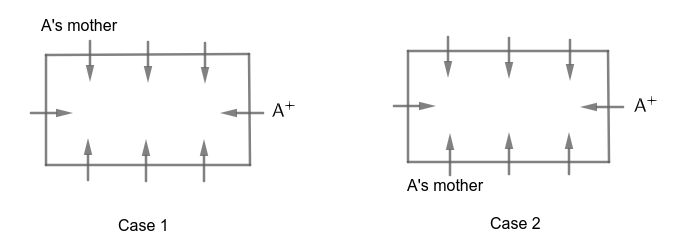A’s aunt is an immediate neighbour of A’s mother. G faces D. Both G and D are males.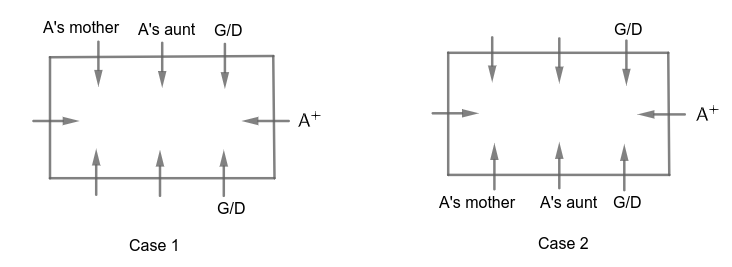G’s sister sits third to the left of G. H sits immediately to the right of his wife. H’s wife is not an immediate neighbour of G.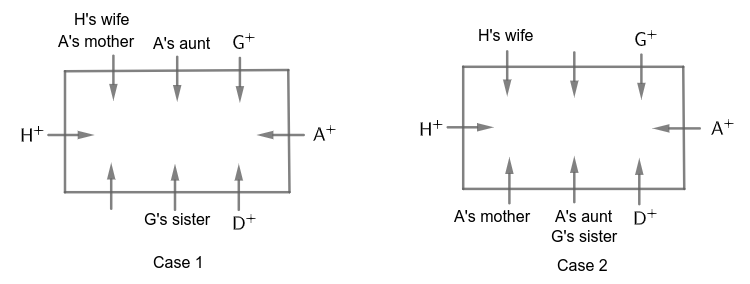H is the father of E. B is the only daughter of H. So E is the son of H and E’s only sister is B.
Three people sit between E and E’s sister. E and E’s sister is not possible in Case 1. Hence Case 1 is eliminated.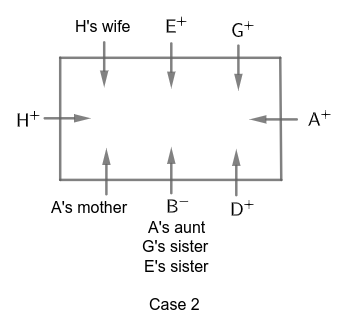F sits immediate left of B. Three people sit between C and C’s son in Law.
The final arrangement is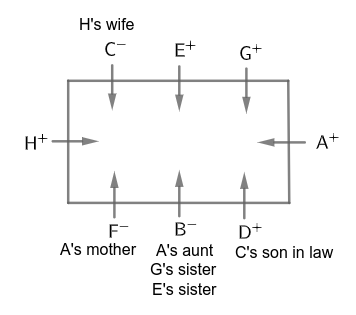E is not married. D do not have siblings.
In the family tree,
= denotes for wife and husband
$+$ and $-$ denotes male and female respectively
Lines are denoted for children
According to the problem, the family tree is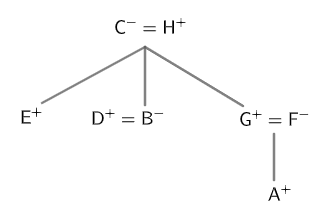Hence, the correct answer is Option D

Question 19: Four of the following are like in a certain way based on pattern and form a group. Which of the following do not belong to the group?

a) G

b) H

c) F

d) E

e) A

Solution:

A sits on the shorter side of the table. Two people sit between A and A’s mother. The two possibilities areA’s aunt is an immediate neighbour of A’s mother. G faces D. Both G and D are males.G’s sister sits third to the left of G. H sits immediately to the right of his wife. H’s wife is not an immediate neighbour of G.H is the father of E. B is the only daughter of H. So E is the son of H and E’s only sister is B.
Three people sit between E and E’s sister. E and E’s sister is not possible in Case 1. Hence Case 1 is eliminated.F sits immediate left of B. Three people sit between C and C’s son in Law.
The final arrangement isE is not married. D do not have siblings.
In the family tree,
= denotes for wife and husband
$+$ and $-$ denotes male and female respectively
Lines are denoted for children
According to the problem, the family tree isExcept F, all the given people are males.
Hence, the correct answer is Option C

Question 20: How many people sit between B and C when counted from the left of C?

a) Two

b) Four

c) One

d) None

e) Three

Solution:

A sits on the shorter side of the table. Two people sit between A and A’s mother. The two possibilities areA’s aunt is an immediate neighbour of A’s mother. G faces D. Both G and D are males.G’s sister sits third to the left of G. H sits immediately to the right of his wife. H’s wife is not an immediate neighbour of G.H is the father of E. B is the only daughter of H. So E is the son of H and E’s only sister is B.
Three people sit between E and E’s sister. E and E’s sister is not possible in Case 1. Hence Case 1 is eliminated.F sits immediate left of B. Three people sit between C and C’s son in Law.
The final arrangement isE is not married. D do not have siblings.
In the family tree,
= denotes for wife and husband
$+$ and $-$ denotes male and female respectively
Lines are denoted for children
According to the problem, the family tree is$\therefore$ Four people sit between B and C when counted from the left of C.
Hence, the correct answer is Option B